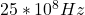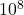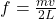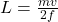## The width of a particular microwave oven is exactly right to support a standing-wave mode. Measurements of the temperature across the oven s

Question

The width of a particular microwave oven is exactly right to support a standing-wave mode. Measurements of the temperature across the oven show that there are cold spots at each edge of the oven and at three spots in between. The wavelength of the microwaves is 12 cm.
a. How wide is the oven?b. What is the frequency of the microwaves?

in progress 0
2 weeks 2021-09-01T12:10:42+00:00 1 Answers 0 views 0

a. The oven is 24.0 cm wide

b. The frequency of the microwaves isExplanation:

Use wavelength of microwave to calculate frequency

f = c / λ

f = ( 3.0 x) / 12

f = 25 xHz

Relationship between frequency of wave and length of wave==>Substituting for m, v, f

L = 0.24m

∴ L = 24cm